# 3.6: Interpreting Correlations

$$\newcommand{\vecs}{\overset { \rightharpoonup} {\mathbf{#1}} }$$ $$\newcommand{\vecd}{\overset{-\!-\!\rightharpoonup}{\vphantom{a}\smash {#1}}}$$$$\newcommand{\id}{\mathrm{id}}$$ $$\newcommand{\Span}{\mathrm{span}}$$ $$\newcommand{\kernel}{\mathrm{null}\,}$$ $$\newcommand{\range}{\mathrm{range}\,}$$ $$\newcommand{\RealPart}{\mathrm{Re}}$$ $$\newcommand{\ImaginaryPart}{\mathrm{Im}}$$ $$\newcommand{\Argument}{\mathrm{Arg}}$$ $$\newcommand{\norm}{\| #1 \|}$$ $$\newcommand{\inner}{\langle #1, #2 \rangle}$$ $$\newcommand{\Span}{\mathrm{span}}$$ $$\newcommand{\id}{\mathrm{id}}$$ $$\newcommand{\Span}{\mathrm{span}}$$ $$\newcommand{\kernel}{\mathrm{null}\,}$$ $$\newcommand{\range}{\mathrm{range}\,}$$ $$\newcommand{\RealPart}{\mathrm{Re}}$$ $$\newcommand{\ImaginaryPart}{\mathrm{Im}}$$ $$\newcommand{\Argument}{\mathrm{Arg}}$$ $$\newcommand{\norm}{\| #1 \|}$$ $$\newcommand{\inner}{\langle #1, #2 \rangle}$$ $$\newcommand{\Span}{\mathrm{span}}$$$$\newcommand{\AA}{\unicode[.8,0]{x212B}}$$

What does the presence or the absence of a correlation between two measures mean? How should correlations be interpreted? What kind of inferences can be drawn from correlations? These are all very good questions. A first piece of advice is to use caution when interpreting correlations. Here’s why.

## Correlation does not equal causation

Perhaps you have heard that correlation does not equal causation. Why not? There are lots of reasons why not. However, before listing some of the reasons let’s start with a case where we would expect a causal connection between two measurements. Consider, buying a snake plant for your home. Snake plants are supposed to be easy to take care of because you can mostly ignore them.

Like most plants, snake plants need some water to stay alive. However, they also need just the right amount of water. Imagine an experiment where 1000 snake plants were grown in a house. Each snake plant is given a different amount of water per day, from zero teaspoons of water per day to 1000 teaspoons of water per day. We will assume that water is part of the causal process that allows snake plants to grow. The amount of water given to each snake plant per day can also be one of our measures. Imagine further that every week the experimenter measures snake plant growth, which will be the second measurement. Now, can you imagine for yourself what a scatter plot of weekly snake plant growth by tablespoons of water would look like?

### Even when there is causation, there might not be obvious correlation

The first plant given no water at all would have a very hard time and eventually die. It should have the least amount of weekly growth. How about the plants given only a few teaspoons of water per day. This could be just enough water to keep the plants alive, so they will grow a little bit but not a lot. If you are imagining a scatter plot, with each dot being a snake plant, then you should imagine some dots starting in the bottom left hand corner (no water & no plant growth), moving up and to the right (a bit of water, and a bit of growth). As we look at snake plants getting more and more water, we should see more and more plant growth, right? “Sure, but only up to a point”. Correct, there should be a trend for a positive correlation with increasing plant growth as amount of water per day increases. But, what happens when you give snake plants too much water? From personal experience, they die. So, at some point, the dots in the scatter plot will start moving back down again. Snake plants that get way too much water will not grow very well.

The imaginary scatter plot you should be envisioning could have an upside U shape. Going from left to right, the dot’s go up, they reach a maximum, then they go down again reaching a minimum. Computing Pearson’s $$r$$ for data like this can give you $$r$$ values close to zero. The scatter plot could look something like this: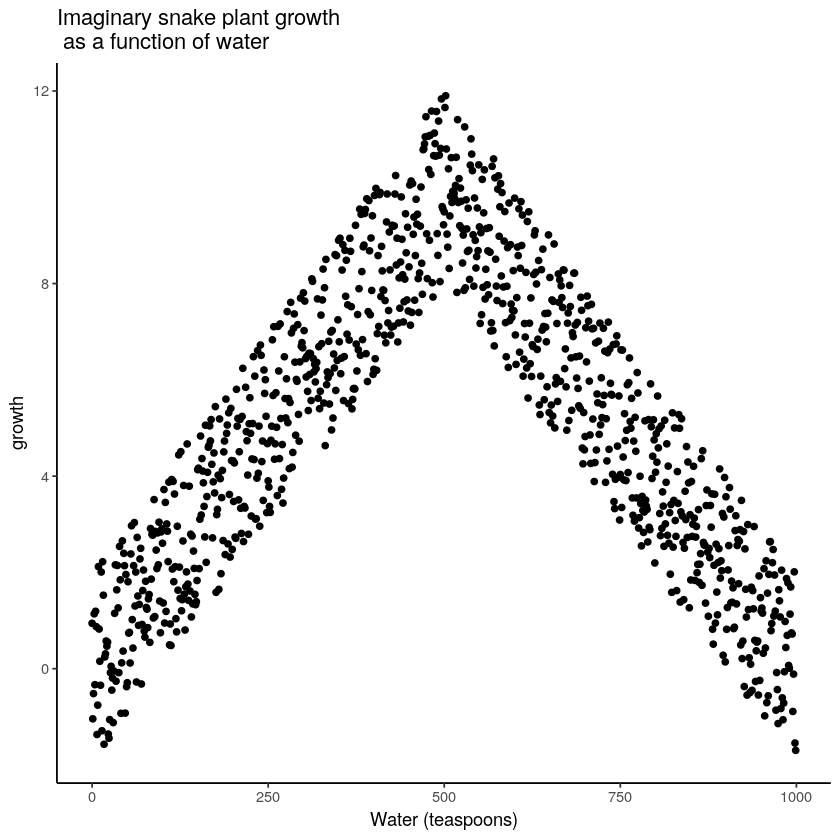Figure $$\PageIndex{1}$$: Illustration of a possible relationship between amount of water and snake plant growth. Growth goes up with water, but eventually goes back down as too much water makes snake plants die.

Granted this looks more like an inverted V, than an inverted U, but you get the picture right? There is clearly a relationship between watering and snake plant growth. But, the correlation isn’t in one direction. As a result, when we compute the correlation in terms of Pearson’s r, we get a value suggesting no relationship.

water<-seq(0,999,1)
growth<-c(seq(0,10,(10/499)),seq(10,0,-(10/499)))
noise<-runif(1000,-2,2)
growth<-growth+noise
cor(growth,water)
-0.0051489425461363

What this really means is there is no linear relationship that can be described by a single straight line. When we need lines or curves going in more than one direction, we have a nonlinear relationship.

This example illustrates some conundrums in interpreting correlations. We already know that water is needed for plants to grow, so we are rightly expecting there to be a relationship between our measure of amount of water and plant growth. If we look at the first half of the data we see a positive correlation, if we look at the last half of the data we see a negative correlation, and if we look at all of the data we see no correlation. Yikes. So, even when there is a causal connection between two measures, we won’t necessarily obtain clear evidence of the connection just by computing a correlation coefficient.

Pro Tip: This is one reason why plotting your data is so important. If you see an upside U shape pattern, then a correlation analysis is probably not the best analysis for your data.

### Confounding variable, or Third variable problem

Anybody can correlate any two things that can be quantified and measured. For example, we could find a hundred people, ask them all sorts of questions like:

1. how happy are you
2. how old are you
3. how tall are you
4. how much money do you make per year
5. how long are your eyelashes
7. how loud is your inner voice

Let’s say we found a positive correlation between yearly salary and happiness. Note, we could have just as easily computed the same correlation between happiness and yearly salary. If we found a correlation, would you be willing to infer that yearly salary causes happiness? Perhaps it does play a small part. But, something like happiness probably has a lot of contributing causes. Money could directly cause some people to be happy. But, more likely, money buys people access to all sorts of things, and some of those things might contribute happiness. These “other” things are called third variables. For example, perhaps people living in nicer places in more expensive houses are more happy than people in worse places in cheaper houses. In this scenario, money isn’t causing happiness, it’s the places and houses that money buys. But, even is this were true, people can still be more or less happy in lots of different situations.

The lesson here is that a correlation can occur between two measures because of a third variable that is not directly measured. So, just because we find a correlation, does not mean we can conclude anything about a causal connection between two measurements.

## Correlation and Random chance

Another very important aspect of correlations is the fact that they can be produced by random chance. This means that you can find a positive or negative correlation between two measures, even when they have absolutely nothing to do with one another. You might have hoped to find zero correlation when two measures are totally unrelated to each other. Although this certainly happens, unrelated measures can accidentally produce spurious correlations, just by chance alone.

Let’s demonstrate how correlations can occur by chance when there is no causal connection between two measures. Imagine two participants. One is at the North pole with a lottery machine full of balls with numbers from 1 to 10. The other is at the south pole with a different lottery machine full of balls with numbers from 1 to 10. There are an endless supply of balls in the machine, so every number could be picked for any ball. Each participant randomly chooses 10 balls, then records the number on the ball. In this situation we will assume that there is no possible way that balls chosen by the first participant could causally influence the balls chosen by the second participant. They are on the other side of the world. We should assume that the balls will be chosen by chance alone.

Here is what the numbers on each ball could look like for each participant:

Ball<-1:10
North_pole<-round(round(runif(10,1,10)))
South_pole<-round(round(runif(10,1,10)))
the_df_balls<-data.frame(Ball,North_pole,South_pole)
#the_df_balls <- the_df_balls %>%
#  rbind(c("Sums",colSums(the_df_balls[1:10,2:3]))) %>%
#  rbind(c("Means",colMeans(the_df_balls[1:10,2:3])))
knitr::kable(the_df_balls)
Ball North_pole South_pole
1 3 1
2 7 7
3 8 8
4 6 9
5 4 6
6 10 10
7 2 2
8 5 8
9 2 5
10 2 3

In this one case, if we computed Pearson's $$r$$, we would find that $$r =$$

North_pole<-round(round(runif(10,1,10)))
South_pole<-round(round(runif(10,1,10)))
cor(North_pole,South_pole)
0.0803444730711034

But, we already know that this value does not tell us anything about the relationship between the balls chosen in the north and south pole. We know that relationship should be completely random, because that is how we set up the game.

The better question here is to ask what can random chance do? For example, if we ran our game over and over again thousands of times, each time choosing new balls, and each time computing the correlation, what would we find? First, we will find fluctuation. The r value will sometimes be positive, sometimes be negative, sometimes be big and sometimes be small. Second, we will see what the fluctuation looks like. This will give us a window into the kinds of correlations that chance alone can produce. Let's see what happens.

### Monte-carlo simulation of random correlations

It is possible to use a computer to simulate our game as many times as we want. This process is often termed monte-carlo simulation.

Below is a script written for the programming language R. We won't go into the details of the code here. However, let's briefly explain what is going on. Notice, the part that says for(sim in 1:1000). This creates a loop that repeats our game 1000 times. Inside the loop there are variables named North_pole and South_pole. During each simulation, we sample 10 random numbers (between 1 to 10) into each variable. These random numbers stand for the numbers that would have been on the balls from the lottery machine. Once we have 10 random numbers for each, we then compute the correlation using cor(North_pole,South_pole). Then, we save the correlation value and move on to the next simulation. At the end, we will have 1000 individual Pearson $$r$$ values.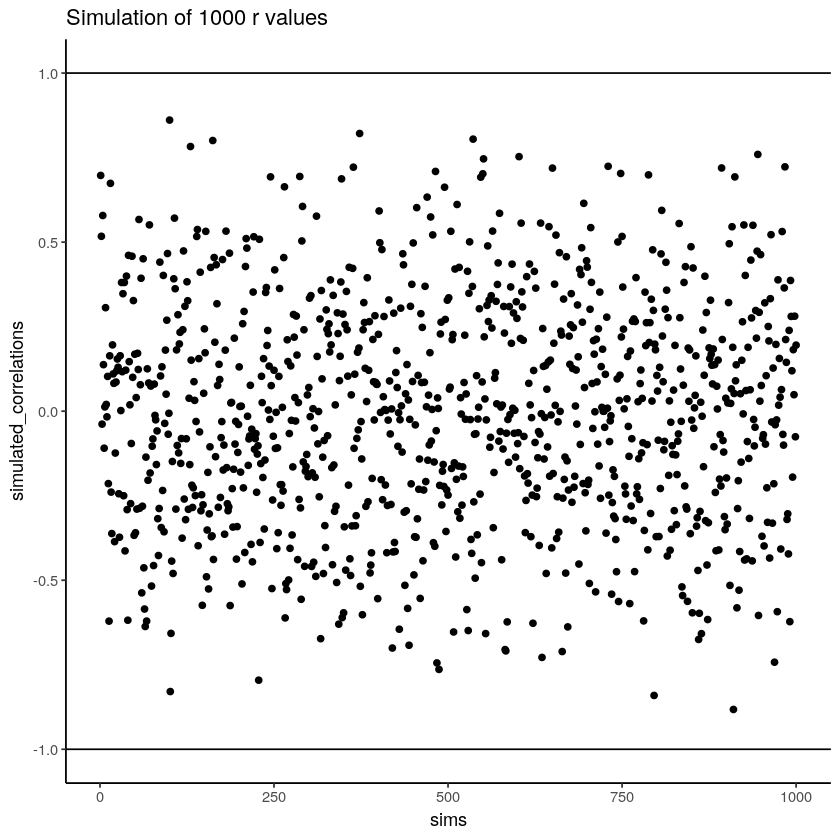Figure $$\PageIndex{2}$$: Another figure showing a range of r-values that can be obtained by chance.

Let’s take a look at all of the 1000 Pearson $$r$$ values. Does the figure below look familiar to you? It should, we have already conducted a similar kind of simulation before. Each dot in the scatter plot shows the Pearson $$r$$ for each simulation from 1 to 1000. As you can see the dots are all over of the place, in between the range -1 to 1. The important lesson here is that random chance produced all of these correlations. This means we can find “correlations” in the data that are completely meaningless, and do not reflect any causal relationship between one measure and another.

Let’s illustrate the idea of finding “random” correlations one more time, with a little movie. This time, we will show you a scatter plot of the random values sampled for the balls chosen from the North and South pole. If there is no relationship we should see dots going everywhere. If there happens to be a positive relationship (purely by chance), we should see the dots going from the bottom left to the top right. If there happens to be a negative relationship (purely by chance), we should see the dots going from the top left down to the bottom right.

On more thing to prepare you for the movie. There are three scatter plots below, showing negative, positive, and zero correlations between two variables. You’ve already seen this graph before. We are just reminding you that the blue lines are helpful for seeing the correlation.Negative correlations occur when a line goes down from the top left to bottom right. Positive correlations occur when a line goes up from the bottom left to the top right. Zero correlations occur when the line is flat (doesn’t go up or down).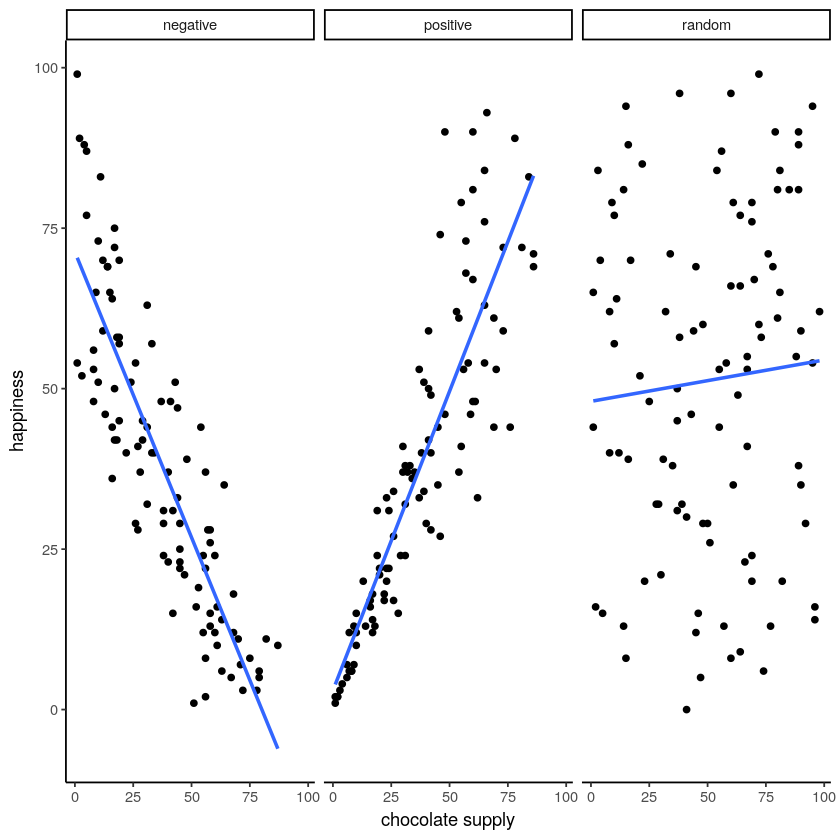Figure $$\PageIndex{3}$$: A reminder of what positive, negative, and zero correlation looks like.

OK, now we are ready for the movie. You are looking at the process of sampling two sets of numbers randomly, one for the X variable, and one for the Y variable. Each time we sample 10 numbers for each, plot them, then draw a line through them. Remember, these numbers are all completely random, so we should expect, on average that there should be no correlation between the numbers. However, this is not what happens. You can the line going all over the place. Sometimes we find a negative correlation (line goes down), sometimes we see a positive correlation (line goes up), and sometimes it looks like zero correlation (line is more flat).Figure $$\PageIndex{4}$$: Completely random data points drawn from a uniform distribution with a small sampl-size of 10. The blue line twirls around sometimes showing large correlations that are produced by chance.

You might be thinking this is kind of disturbing. If we know that there should be no correlation between two random variables, how come we are finding correlations? This is a big problem right? I mean, if someone showed me a correlation between two things, and then claimed one thing was related to another, how could know I if it was true. After all, it could be chance! Chance can do that too.

Fortunately, all is not lost. We can look at our simulated data in another way, using a histogram. Remember, just before the movie, we simulated 1000 different correlations using random numbers. By, putting all of those $$r$$ values into a histogram, we can get a better sense of how chance behaves. We can see what kind of correlations chance is likely or unlikely to produce. Here is a histogram of the simulated $$r$$ values.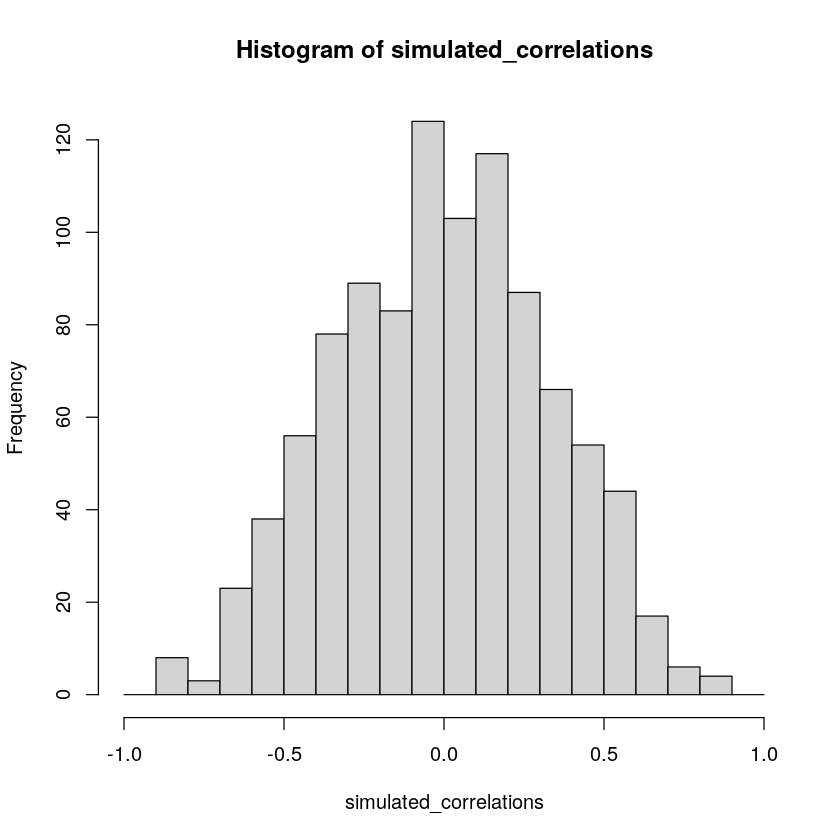Figure $$\PageIndex{5}$$: A histogram showing the frequency distribution of $$r$$-values for completely random values between an X and Y variable (sample-size=10). A rull range of $$r$$-values can be obtained by chance alone. Larger $$r$$-values are less common than smaller $$r$$-values.

Notice that this histogram is not flat. Most of the simulated $$r$$ values are close to zero. Notice, also that the bars get smaller as you move away from zero in the positive or negative direction. The general take home here is that chance can produce a wide range of correlations. However, not all correlations happen very often. For example, the bars for -1 and 1 are very small. Chance does not produce nearly perfect correlations very often. The bars around -.5 and .5 are smaller than the bars around zero, as medium correlations do not occur as often as small correlations by chance alone.

You can think of this histogram as the window of chance. It shows what chance often does, and what it often does not do. If you found a correlation under these very same circumstances (e.g., measured the correlation between two sets of 10 random numbers), then you could consult this window. What should you ask the window? How about, could my observed correlation (the one that you found in your data) have come from this window. Let’s say you found a correlation of $$r = .1$$. Could a .1 have come from the histogram? Well, look at the histogram around where the .1 mark on the x-axis is. Is there a big bar there? If so, this means that chance produces this value fairly often. You might be comfortable with the inference: Yes, this .1 could have been produced by chance, because it is well inside the window of chance. How about $$r = .5$$? The bar is much smaller here, you might think, “well, I can see that chance does produce .5 some times, so chance could have produced my .5. Did it? Maybe, maybe not, not sure”. Here, your confidence in a strong inference about the role of chance might start getting a bit shakier.

How about an $$r = .95$$?. You might see that the bar for .95 is very very small, perhaps too small to see. What does this tell you? It tells you that chance does not produce .95 very often, hardly if at all, pretty much never. So, if you found a .95 in your data, what would you infer? Perhaps you would be comfortable inferring that chance did not produce your .95, after .95 is mostly outside the window of chance.

### Increasing sample-size decreases opportunity for spurious correlation

Before moving on, let’s do one more thing with correlations. In our pretend lottery game, each participant only sampled 10 balls each. We found that this could lead to a range of correlations between the numbers randomly drawn from either sides of the pole. Indeed, we even found some correlations that were medium to large in size. If you were a researcher who found such correlations, you might be tempted to believe there was a relationship between your measurements. However, we know in our little game, that those correlations would be spurious, just a product of random sampling.

The good news is that, as a researcher, you get to make the rules of the game. You get to determine how chance can play. This is all a little bit metaphorical, so let’s make it concrete.

We will see what happens in four different scenarios. First, we will repeat what we already did. Each participant will draw 10 balls, then we compute the correlation, and do this over 1000 times and look at a histogram. Second, we will change the game so each participant draws 50 balls each, and then repeat our simulation. Third, and fourth, we will change the game so each participant draws 100 balls each, and then 1000 balls each, and repeat etc.

The graph below shows four different histograms of the Pearson $$r$$ values in each of the different scenarios. Each scenario involves a different sample-size, from, 10, 50, 100 to 1000.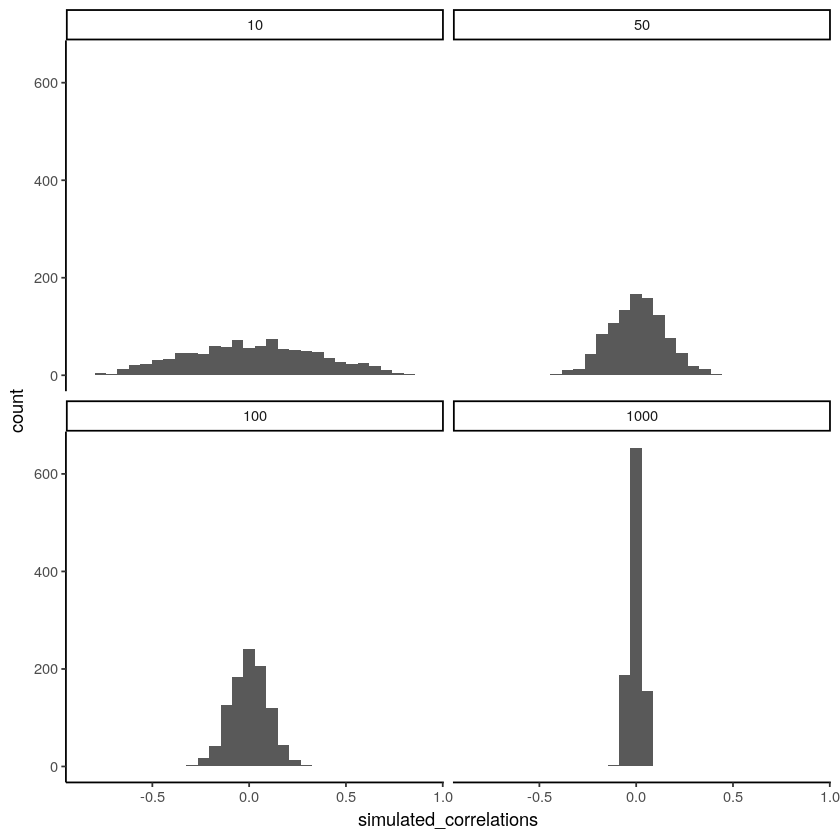Figure $$\PageIndex{6}$$: Four histograms showing the frequency distributions of $$r$$-values between completely random X and Y variables as a function of sample-size. The width of the distributions shrink as sample-size increases. Smaller sample-sizes are more likely to produce a wider range of $$r$$-values by chance. Larger sample-sizes always produce a narrow range of small $$r$$-values.

By inspecting the four histograms you should notice a clear pattern. The width or range of each histogram shrinks as the sample-size increases. What is going on here? Well, we already know that we can think of these histograms as windows of chance. They tell us which $$r$$ values occur fairly often, which do not. When our sample-size is 10, lots of different $$r$$ values happen. That histogram is very flat and spread out. However, as the sample-size increases, we see that the window of chance gets pulled in. For example, by the time we get to 1000 balls each, almost all of the Pearson $$r$$ values are very close to 0.

One take home here, is that increasing sample-size narrows the window of chance. So, for example, if you ran a study involving 1000 samples of two measures, and you found a correlation of .5, then you can clearly see in the bottom right histogram that .5 does not occur very often by chance alone. In fact, there is no bar, because it didn't happen even once in the simulation. As a result, when you have a large sample size like n = 1000, you might be more confident that your observed correlation (say of .5) was not a spurious correlation. If chance is not producing your result, then something else is.

Finally, notice how your confidence about whether or not chance is mucking about with your results depends on your sample size. If you only obtained 10 samples per measurement, and found $$r = .5$$, you should not be as confident that your correlation reflects a real relationship. Instead, you can see that $$r$$'s of .5 happen fairly often by chance alone.

Pro tip: when you run an experiment you get to decide how many samples you will collect, which means you can choose to narrow the window of chance. Then, if you find a relationship in the data you can be more confident that your finding is real, and not just something that happened by chance.

## Some more movies

Let's ingrain these idea with some more movies. When our sample-size is small (N is small), sampling error can cause all sort "patterns" in the data. This makes it possible, and indeed common, for "correlations" to occur between two sets of numbers. When we increase the sample-size, sampling error is reduced, making it less possible for "correlations" to occur just by chance alone. When N is large, chance has less of an opportunity to operate.

### Watching how correlation behaves when there is no correlation

Below we randomly sample numbers for two variables, plot them, and show the correlation using a line. There are four panels, each showing the number of observations in the samples, from 10, 50, 100, to 1000 in each sample.

Remember, because we are randomly sampling numbers, there should be no relationship between the X and Y variables. But, as we have been discussing, because of chance, we can sometimes observe a correlation (due to chance). The important thing to watch is how the line behaves across the four panels. The line twirls around in all directions when the sample size is 10. It is also moves around quite a bit when the sample size is 50 or 100. It still moves a bit when the sample size is 1000, but much less. In all cases we expect that the line should be flat, but every time we take new samples, sometimes the line shows us pseudo patterns.Figure $$\PageIndex{7}$$: Animation of how correlation behaves for completely random X and Y variables as a function of sample size. The best fit line is not very stable for small sample-sizes, but becomes more reliably flat as sample-size increases.

Which line should you trust? Well, hopefully you can see that the line for 1000 samples is the most stable. It tends to be very flat every time, and it does not depend so much on the particular sample. The line with 10 observations per sample goes all over the place. The take home here, is that if someone told you that they found a correlation, you should want to know how many observations they hand in their sample. If they only had 10 observations, how could you trust the claim that there was a correlation? You can’t!!! Not now that you know samples that are that small can do all sorts of things by chance alone. If instead, you found out the sample was very large, then you might trust that finding a little bit more. For example, in the above movie you can see that when there are 1000 samples, we never see a strong or weak correlation; the line is always flat. This is because chance almost never produces strong correlations when the sample size is very large.

In the above example, we sampled numbers random numbers from a uniform distribution. Many examples of real-world data will come from a normal or approximately normal distribution. We can repeat the above, but sample random numbers from the same normal distribution. There will still be zero actual correlation between the X and Y variables, because everything is sampled randomly. But, we still see the same behavior as above. The computed correlation for small sample-sizes fluctuate wildly, and large sample sizes do not.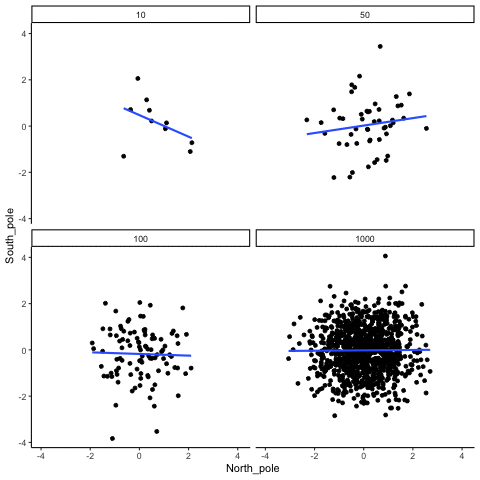Figure $$\PageIndex{8}$$: Animation of correlation for random values sampled from a normal distribution, rather than a uniform distribution.

OK, so what do things look like when there actually is a correlation between variables?

### Watching correlations behave when there really is a correlation

Sometimes there really are correlations between two variables that are not caused by chance. Below, we get to watch a movie of four scatter plots. Each shows the correlation between two variables. Again, we change the sample-size in steps of 10, 50 100, and 1000. The data have been programmed to contain a real positive correlation. So, we should expect that the line will be going up from the bottom left to the top right. However, there is still variability in the data. So this time, sampling error due to chance will fuzz the correlation. We know it is there, but sometimes chance will cause the correlation to be eliminated.

Notice that in the top left panel (sample-size 10), the line is twirling around much more than the other panels. Every new set of samples produces different correlations. Sometimes, the line even goes flat or downward. However, as we increase sample-size, we can see that the line doesn’t change very much, it is always going up showing a positive correlation.Figure $$\PageIndex{9}$$: How correlation behaves as a function of sample-size when there is a true correlation between X and Y variables.

The main takeaway here is that even when there is a positive correlation between two things, you might not be able to see it if your sample size is small. For example, you might get unlucky with the one sample that you measured. Your sample could show a negative correlation, even when the actual correlation is positive! Unfortunately, in the real world we usually only have the sample that we collected, so we always have to wonder if we got lucky or unlucky. Fortunately, if you want to remove luck, all you need to do is collect larger samples. Then you will be much more likely to observe the real pattern, rather the pattern that can be introduced by chance.

This page titled 3.6: Interpreting Correlations is shared under a CC BY-SA 4.0 license and was authored, remixed, and/or curated by Matthew J. C. Crump via source content that was edited to the style and standards of the LibreTexts platform; a detailed edit history is available upon request.